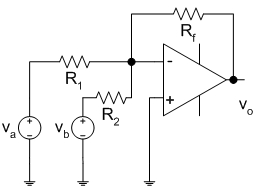EE 212 Lab

Prelab 7: Operational Amplifiers - Part 1

1. For the inverting, summing amplifier shown, find the voltage gain relationship (i.e., relationship between         output vo and inputs va, vb).

1.2. For the circuit of figure 3 in this lab:

a) Find the input impedance to the right of V1.
1.

b)  What is the output impedance of the amplifier circuit?

3. For the circuit of figure 4 in this lab:

1. a) What is the voltage gain of this circuit?

b) What is the input impedance of this circuit?

c)  What is its output impedance of this circuit?